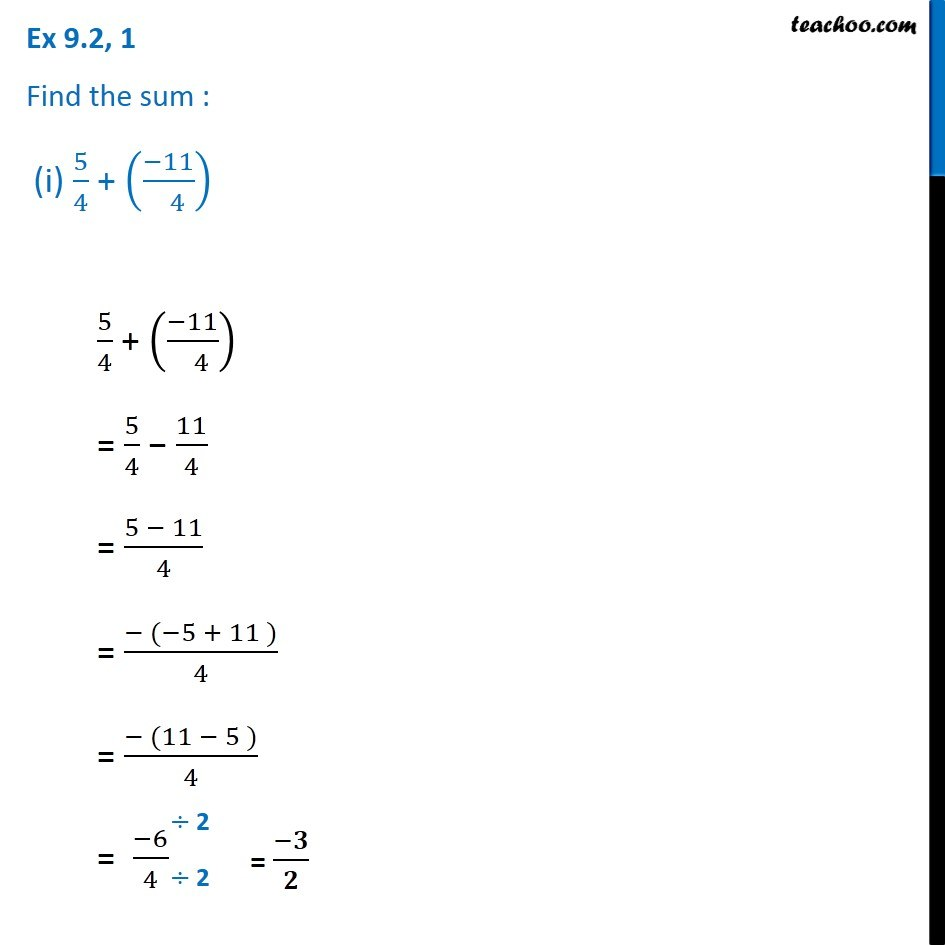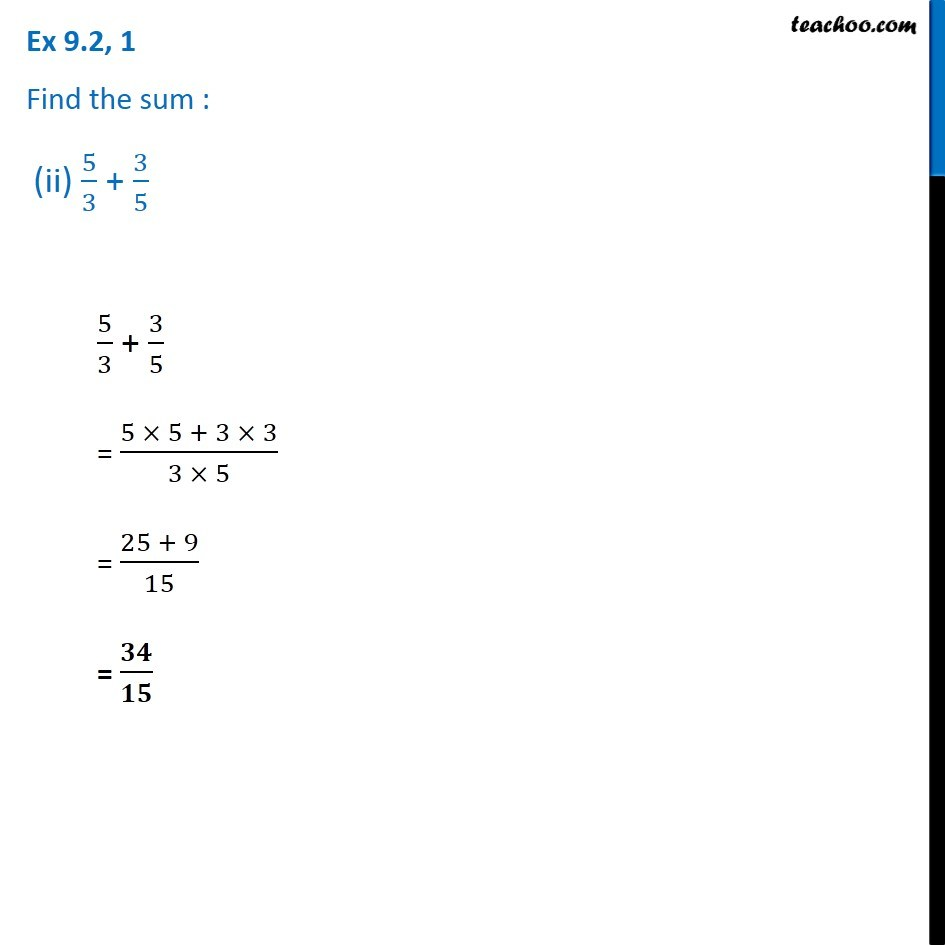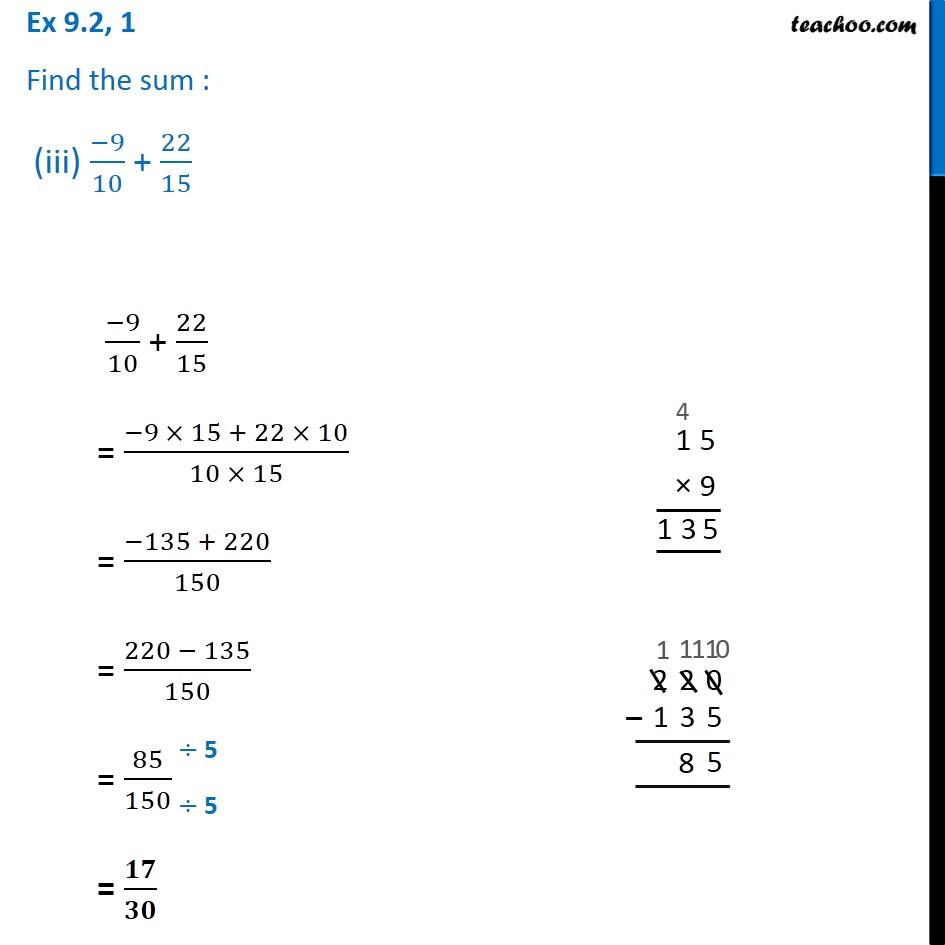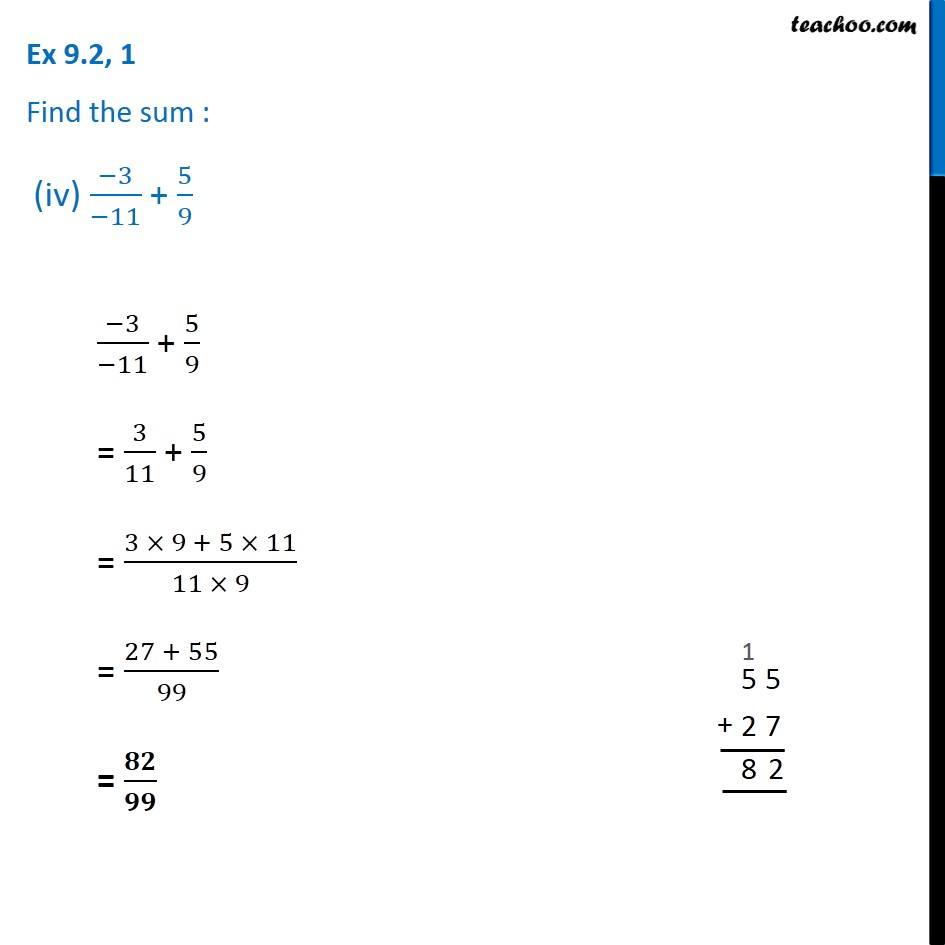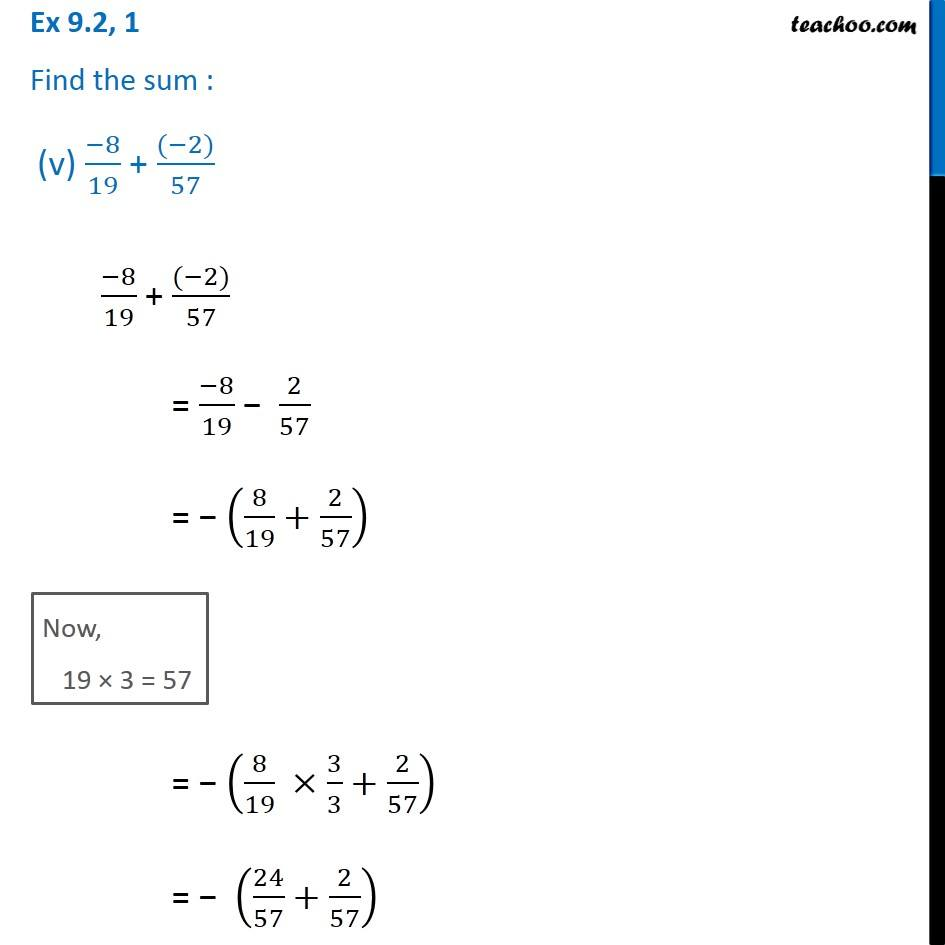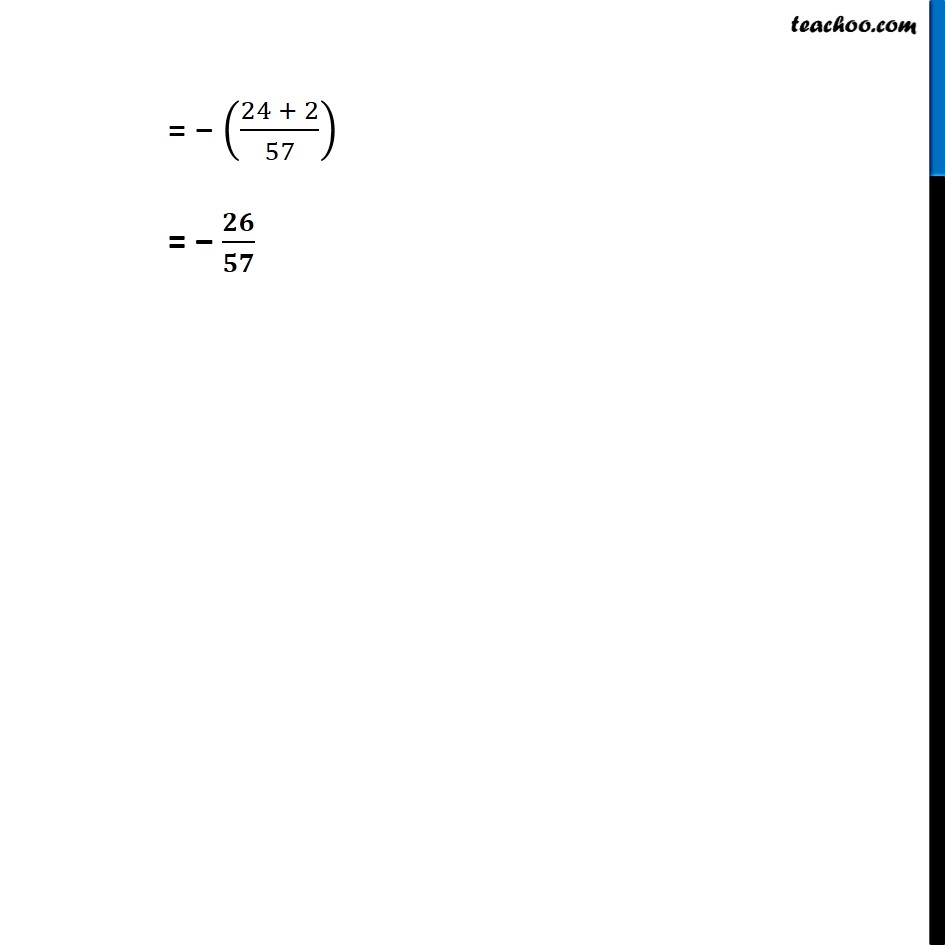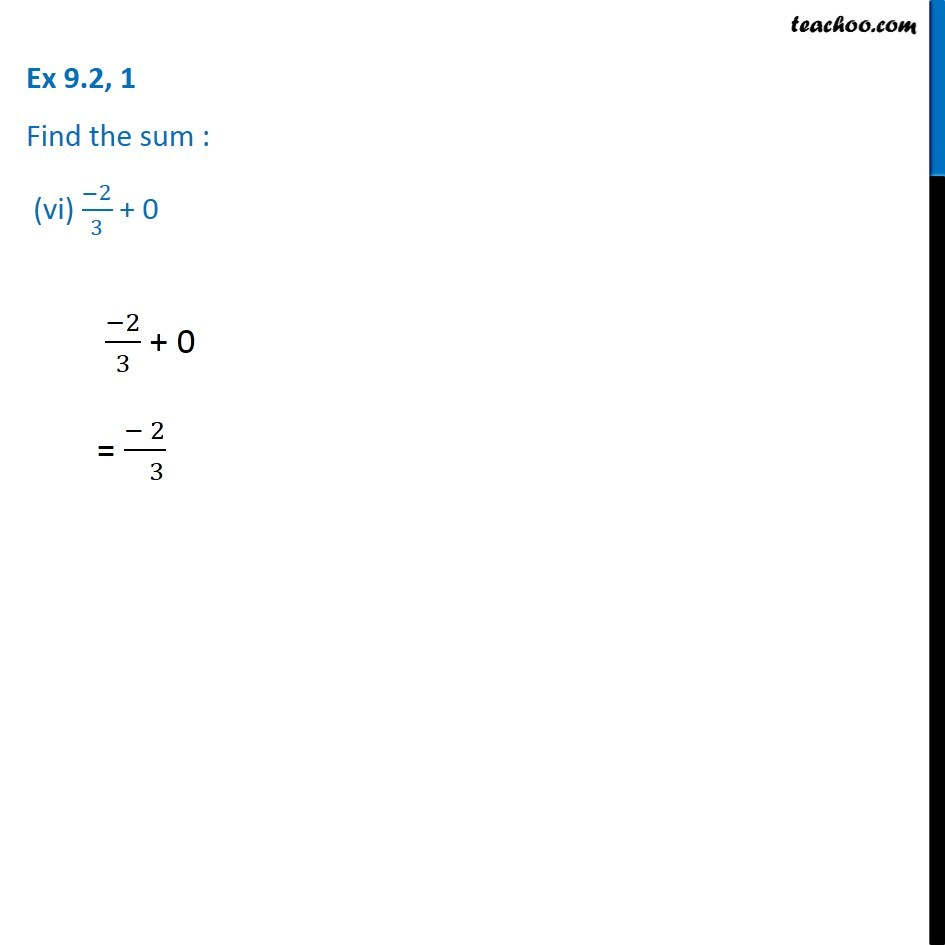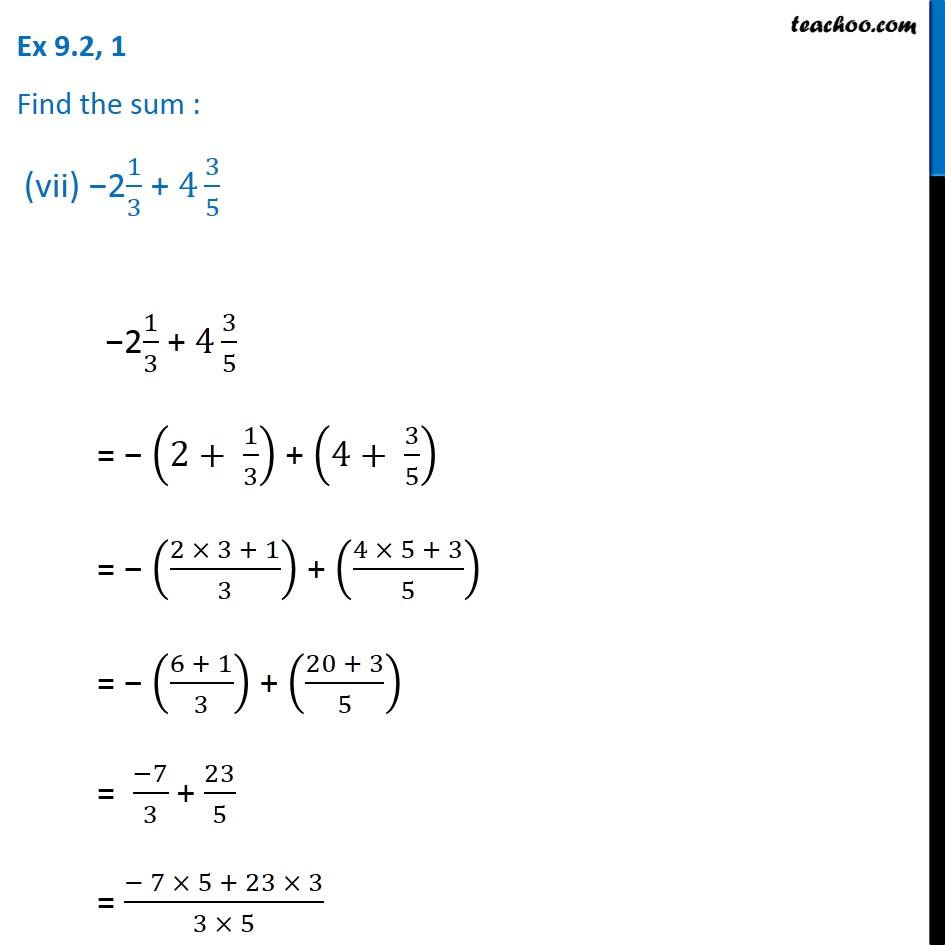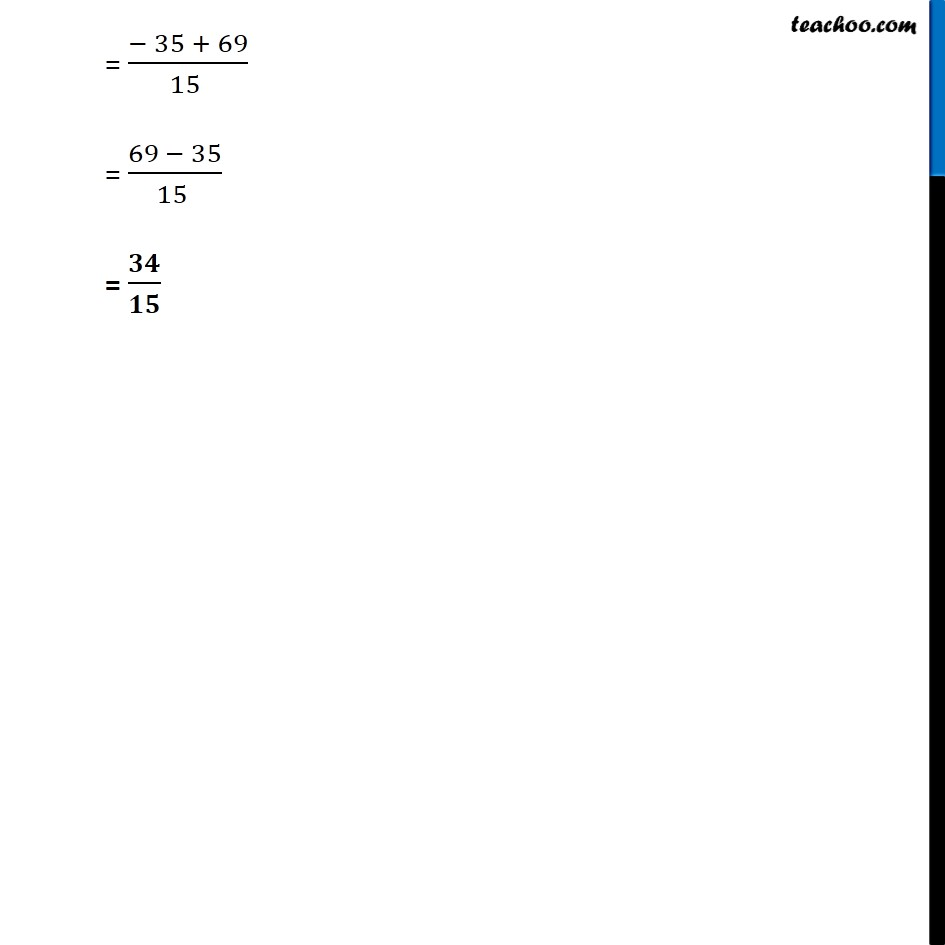1. Chapter 9 Class 7 Rational Numbers
2. Concept wise
3. Addition & Subtraction of rational numbers

Transcript

Ex 9.2, 1 Find the sum : (i) 5/4 + ((−11)/( 4)) 5/4 + ((−11)/( 4)) = 5/4 − 11/4 = (5 − 11)/4 = (− (−5 + 11 ))/4 = (− (11 − 5 ))/4 = (−6)/4 Ex 9.2, 1 Find the sum : (ii) 5/3 + 3/5 5/3 + 3/5 = (5 × 5 + 3 × 3)/(3 × 5) = (25 + 9)/15 = 𝟑𝟒/𝟏𝟓 Ex 9.2, 1 Find the sum : (iii) (−9)/10 + 22/15 (−9)/10 + 22/15 = (−9 × 15 + 22 × 10)/(10 × 15) = (−135 + 220)/150 = (220 − 135)/150 = 85/150 = 𝟏𝟕/𝟑𝟎 Ex 9.2, 1 Find the sum : (iv) (−3)/(−11) + 5/9 (−3)/(−11) + 5/9 = 3/11 + 5/9 = (3 × 9 + 5 × 11)/(11 × 9) = (27 + 55)/99 = 𝟖𝟐/𝟗𝟗 Ex 9.2, 1 Find the sum : (v) (−8)/19 + ((−2))/57 (−8)/19 + ((−2))/57 = (−8)/19 − 2/57 = − (8/19+2/57) Now, 19 × 3 = 57 = − (8/19 ×3/3+2/57) = − (24/57+2/57) = − ((24 + 2)/57) = − 𝟐𝟔/𝟓𝟕 Ex 9.2, 1 Find the sum : (vi) (−2)/3 + 0 (−2)/3 + 0 = (− 2)/( 3) Ex 9.2, 1 Find the sum : (vii) −21/3 + 4 3/5 −21/3 + 4 3/5 = − (2+ 1/3) + (4+ 3/5) = − ((2 × 3 + 1)/3) + ((4 × 5 + 3)/5) = − ((6 + 1)/3) + ((20 + 3)/5) = (−7)/3 + 23/5 = (− 7 × 5 + 23 × 3)/(3 × 5) = (− 35 + 69)/(15 ) = (69 − 35)/(15 ) = 𝟑𝟒/𝟏𝟓

Addition & Subtraction of rational numbers